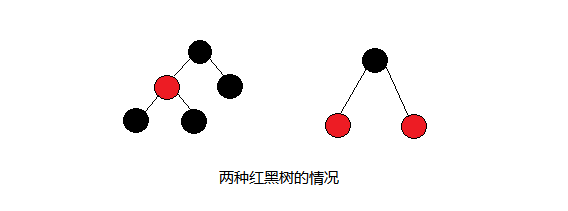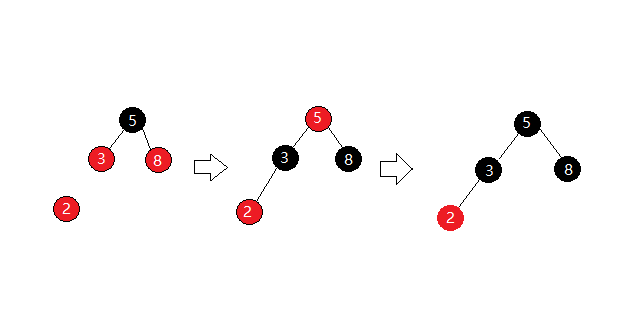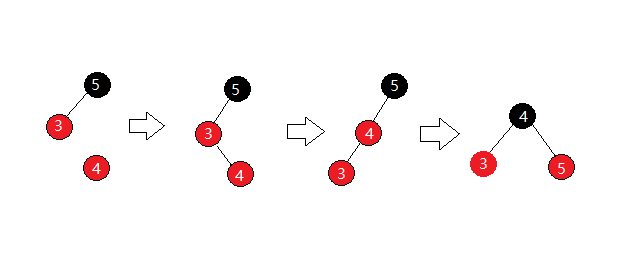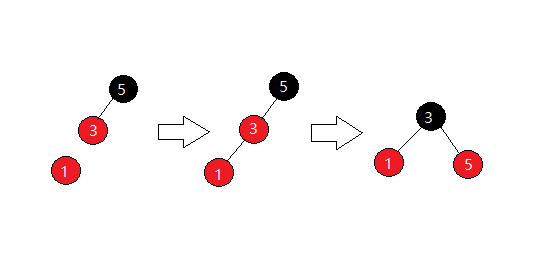# 简介

## 在STL深度剖析中给出了四个规则：

1.结点不是红色就是黑色；
2.根结点是黑色；
3.如果结点是红色的，那么它的两个孩子要为黑色；
4.任一结点到NULL结点即尾结点的任何路径，经过黑色结点的个数相同。# RBTree与AVLTree以及普通二叉搜索树的区别：

## AVLTree：

AVL树是高度平衡的二叉搜索树，在AVL树中任何结点的两个子树的高度差都不超过一，所以其各类操作的时间复杂度都为O（log2N）。这样就大大的降低了二叉搜索树的平均搜索长度，但是为了保持这种结构，不得不在每次插入或者是删除之后对树进行调整。

## RBTree：

RBTree的平衡性没有AVLTree树的高，它只能保证每个结点的一条子树比另一条子树长不超过两倍。维持了基本的平衡状态，所以它的各类操作时间复杂度也为O（log2N）。这样就降低了AVLTree中的调整次数，提高了性能，而且平均搜索长度也和AVLTree相当。

# 结点定义

RBTree的结构和AVLTree差不多，不同的是没有了 _bf (平衡因子) ，取而代之的是_col元素（颜色），用来确定结点的颜色，用它来维持树的基本平衡。

enum Colour
{
RED,
BLACK,
};

template<class K,class V>
struct RBTreeNode
{
K _key;
V _value;
Colour _col;

RBTreeNode<K, V>* _left;
RBTreeNode<K, V>* _right;
RBTreeNode<K, V>* _parent;

RBTreeNode(const K& key, const V& value)
:_key(key)
, _value(value)
, _col(RED)
, _left(NULL)
, _right(NULL)
, _parent(NULL)
{}
};

# 插入操作

1.当前结点的父结点为红，且叔叔结点也为红；
2.当前结点的父结点为红，叔叔结点为黑或者不存在，当前结点是其父结点的右孩子；
3.当前节点的父结点为红，叔叔结点为黑护着不存在，当前结点是其父结点的左孩子；

## 1.父结点为红，且叔叔结点也为红；## 2.父结点为红，叔结点为黑或者不存在，当前结点在右孩子。## 3.父结点为红，叔结点为黑或者不存在，当前结点在左孩子。## 代码实现

bool Insert(const K& key, const V& value)
{//插入
if (_root == NULL)
{
_root = new Node(key, value);
_root->_col = BLACK;
return make_pair(_root, true);
}

Node* cur = _root;
Node* parent = NULL;
while (cur)
{//找到要插入的位置
if (key > cur->_key)
{
parent = cur;
cur = cur->_right;
}
else if (key < cur->_key)
{
parent = cur;
cur = cur->_left;
}
else
return false;
}
//插入结点
cur = new Node(key, value);
if (key > parent->_key)
{
parent->_right = cur;
cur->_parent = parent;
}
else
{
parent->_left = cur;
cur->_parent = parent;
}

while (parent&&parent->_col == RED)
{//父节点为红
Node* grandfather = parent->_parent;
if (grandfather->_left == parent)
{//父节点为左孩子
Node* uncle = grandfather->_right;

if (uncle && uncle->_col == RED)
{//叔结点存在且为红
parent->_col = uncle->_col = BLACK;
grandfather->_col = RED;
cur = grandfather;
parent = cur->_parent;
}
else
{//叔结点不为红或者不存在
if (cur == parent->_right)
{//插入结点为右孩子，先左旋，再调整父子关系
RotateL(parent);
swap(cur, parent);
}
//右旋后调色
RotateR(grandfather);
grandfather->_col = RED;
parent->_col = BLACK;
break;
}
}
else
{//父节点为左孩子，与上面的情况对称
Node* uncle = grandfather->_left;

if (uncle&&uncle->_col == RED)
{
parent->_col = uncle->_col = BLACK;
grandfather->_col = RED;
cur = grandfather;
parent = cur->_parent;
}
else
{
if (cur == parent->_left)
{
RotateR(parent);
swap(cur, parent);
}
else
{
RotateL(grandfather);
parent->_col = BLACK;
grandfather->_col = RED;
}
}
}
}
//根结点置黑
_root->_col = BLACK;

return true;
}

# RBTree的检测

## 代码实现

void IsRBTree()
{//判断是否满足红黑树
if (_root == NULL)
return true;

if (_root->_col == RED)
return false;

size_t count = 0;
Node* cur = _root;
while (cur)
{
if (cur->_col == BLACK)
count++;
cur = cur->_left;
}

size_t num = 0;

return CheckColour(_root) && CheckBlackNum(_root, count, num);
}

bool CheckColour(Node* root)
{//检查颜色
if (root)
return true;

if (root->_col == RED &&root->_parent->_col == RED)
return false;

return CheckColour(root->_left) && CheckColour(root->_right);

}

bool CheckBlackNum(Node* root, const size_t &k, size_t num)
{//检查黑色结点数目
if (root == NULL)
return true;

if (root->_col == BLACK)
++num;

if (root->_left == NULL && root->_right == NULL && num != k)
return false;

return CheckBlackNum(root->_left) && CheckBlackNum(root->_right);
}